# Funds From Operations

In real estate, the Funds From Operations (FFO) and Adjusted Funds From Operations (AFFO) are measures calculated by analysts to value Real Estate Investment Trusts (REITs). While FFO is a good measure of operating income, AFFO is more useful if we want to measure economic income.

On this page, we discuss the (adjusted) funds from operations formula, illustrate the funds from operations calculation, and provide a simple calculator in Excel to help you implement the approach yourself to value a REIT.

## Funds From Operations formula

How to calculate funds from operations? To do that, we have to start from accounting net earnings (NI), and then subtract and add the following elements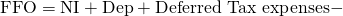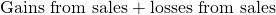where Dep is depreciation, deferred tax expenses consist mainly of deferred tax liabilities and Gains/Losses from sales also include debt restructurings. We add depreciation back because very often, accounting depreciation exceeds economic depreciation. Deferred tax liabilities are also included because they are typically not paid back for many years. Finally, we exclude gains (include losses) from sales and debt restructurings because they are not part of continuing income.

## Adjusted Funds From Operations formula

To calculate AFFO, we start from FFO. We then do the following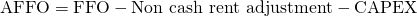Non cash rent or straight line rent refers to the average contractual rent over the lease period. AFFO is also known as Cash Available for Distribution (CAD) or Funds Available for Distribution (FAD).

Now that we discussed both formulas, let’s turn to an example.

## Adjusted Funds From Operations Calculation Example

The following table illustrates how to calculate Funds From Operations (FFO) and adjusted Funds From Operations (AFFO) to value a REIT. Note that we express both FFO and AFFO relative to the stock price of the REIT. Thus, we use multiples to value the REIT.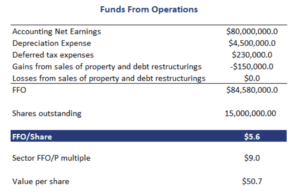Once we have the FFO, we can can calculate AFFO as follows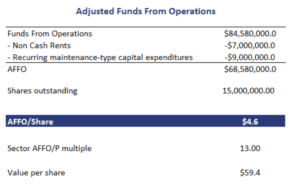## Summary

We discussed two measures to value a REIT; Funds From Operations (FFO) and Adjusted Funds From Operations (AFFO). Both measures are commonly used by analysts because they can typically be performed using the financial statements of a REIT. One other metric that is typically calculated by investors is the funds from operations to debt ratio.